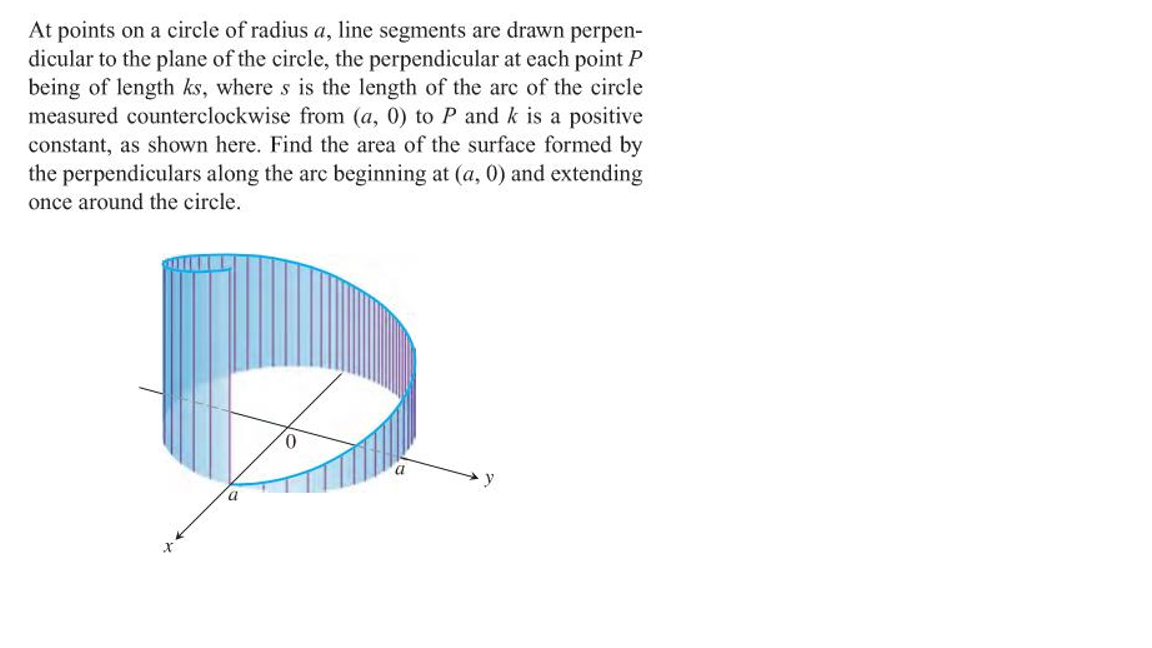# At points on a circle of radius a, line segments are drawn perpen- dicular to the plane of the circle, the perpendicular at each point P being of length ks, where s is the length of the are of the circle measured counterclockwise from (a, 0) to P and k is a positive constant, as shown here. Find the area of the surface formed by the perpendiculars along the arc beginning at (a, 0) and extending once around the circle.

Questionhelp_outlineImage TranscriptioncloseAt points on a circle of radius a, line segments are drawn perpen- dicular to the plane of the circle, the perpendicular at each point P being of length ks, where s is the length of the are of the circle measured counterclockwise from (a, 0) to P and k is a positive constant, as shown here. Find the area of the surface formed by the perpendiculars along the arc beginning at (a, 0) and extending once around the circle. fullscreen

### Want to see this answer and more?

Experts are waiting 24/7 to provide step-by-step solutions in as fast as 30 minutes!*

*Response times may vary by subject and question complexity. Median response time is 34 minutes for paid subscribers and may be longer for promotional offers.
Tagged in
MathCalculus

### Applications of Integration